## RS Aggarwal Class 8 Solutions Chapter 6 Operations on Algebraic Expressions Ex 6C

These Solutions are part of RS Aggarwal Solutions Class 8. Here we have given RS Aggarwal Solutions Class 8 Chapter 6 Operations on Algebraic Expressions Ex 6C.

Other Exercises

Question 1.
Solution:
(i) 24x2y3 ÷ 3xy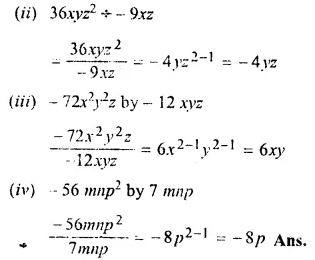Question 2.
Solution:
(i)(5m3 – 30m2 + 45m) ÷ 5m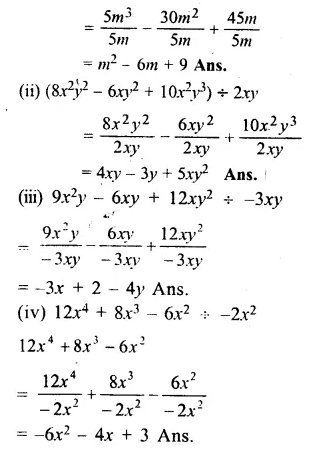Write the quotient and remainder when we divide:

Question 3.
Solution: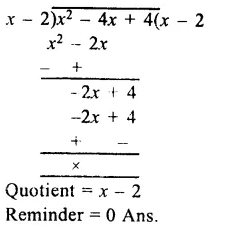Question 4.
Solution: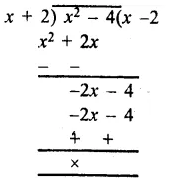Quotient = x – 2
Remainder = 0

Question 5.
Solution:Question 6.
Solution: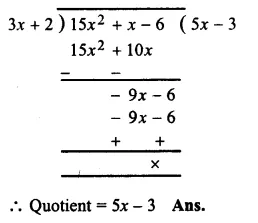Question 7.
Solution:Question 8.
Solution: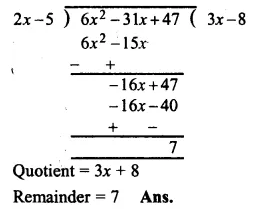Question 9.
Solution: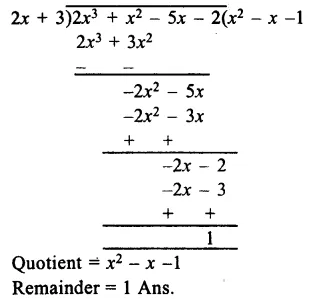Question 10.
Solution: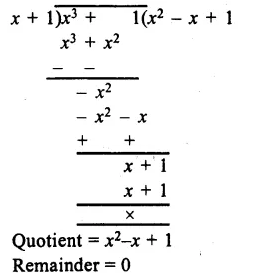Question 11.
Solution: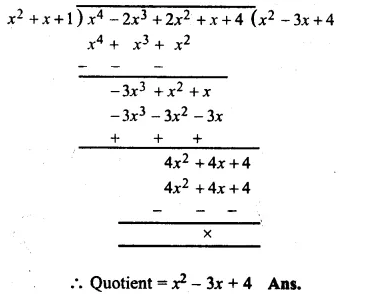Question 12.
Solution: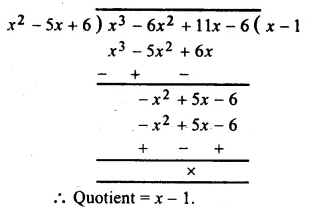Question 13.
Solution:Question 14.
Solution: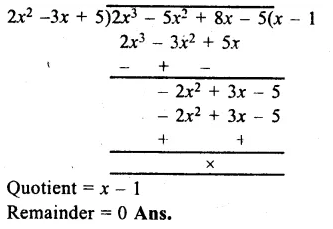Question 15.
Solution: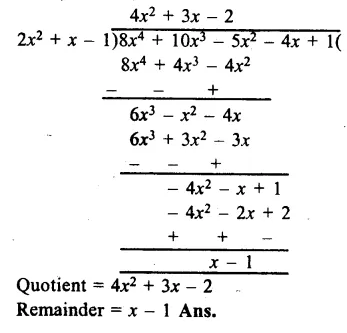Hope given RS Aggarwal Solutions Class 8 Chapter 6 Operations on Algebraic Expressions Ex 6C are helpful to complete your math homework.

If you have any doubts, please comment below. Learn Insta try to provide online math tutoring for you.